# Forces (Part - 3) Civil Engineering (CE) Notes | EduRev

## Civil Engineering (CE) : Forces (Part - 3) Civil Engineering (CE) Notes | EduRev

The document Forces (Part - 3) Civil Engineering (CE) Notes | EduRev is a part of the Civil Engineering (CE) Course Introduction to Dynamics and Vibrations- Notes, Videos, MCQs.
All you need of Civil Engineering (CE) at this link: Civil Engineering (CE)

Hydrostatic and buoyancy forces

When an object is immersed in a stationary fluid, its surface is subjected to a pressure. The pressure is actually induced in the fluid by gravity: the pressure at any depth is effectively supporting the weight of fluid above that depth.

A pressure is a distributed force. If a pressure p acts on a surface, a small piece of the surface with area dA is subjected to a force

dF = -p dA n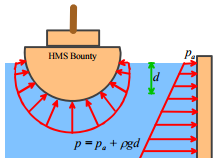where n is a unit vector perpendicular to the surface. The total force on a surface must be calculated by integration. We will show how this is done shortly.

The pressure in a stationary fluid varies linearly with depth below the fluid surface p = pa = pgd

where pa is atmospheric pressure (often neglected as it’s generally small compared with the second term); ρ is the fluid density; g is the acceleration due to gravity; and d is depth below the fluid surface.

Archimedes’ principle gives a simple way to calculate the resultant force exerted by fluid pressure on an immersed object.

The magnitude of the resultant force is equal to the weight of water displaced by the object. The direction is perpendicular to the fluid surface. Thus, if the fluid has mass density ρ , and a volume VI of the object lies below the surface of the fluid, the resultant force due to fluid pressure is

F = ρgVi j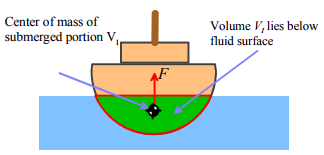The force acts at the center of buoyancy of the immersed object. The center of buoyancy can be calculated by finding the center of mass of the displaced fluid (i.e. the center of mass of the portion of the immersed object that lies below the fluid surface).

The buoyancy force acts in addition to gravity loading. If the object floats, the gravitational force is equal and opposite to the buoyancy force. The force of gravity acts (as usual) at the center of mass of the entire object.

Aerodynamic lift and drag forces

Engineers who design large bridges, buildings, or fast-moving terrestrial vehicles, spend much time and effort in managing aeroor hydro-dynamic forces. Hydrodynamic forces are also of great interest to engineers who design bearings and car tires, since hydrodynamic forces can cause one surface to float above another, so reducing friction to very low levels.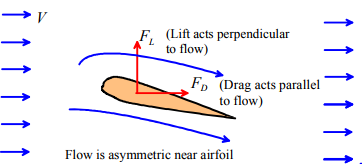In general, when air or fluid flow past an object (or equivalently, if the object moves through stationary fluid or gas), the object is subjected to two forces:

1. A Drag force, which acts parallel to the direction of air or fluid flow
2. A Lift force, which acts perpendicular to the direction of air or fluid flow.

The forces act at a point known as the center of lift of the object – but there’s no simple way to predict where this point is.

The lift force is present only if airflow past the object is unsymmetrical (i.e. faster above or below the object). This asymmetry can result from the shape of the object itself (this effect is exploited in the design of airplane wings); or because the object is spinning (this effect is exploited by people who throw, kick, or hit balls for a living).

Two effects contribute to drag:

(1) Friction between the object’s surface and the fluid or air. The friction force depends on the object’s shape and size; on the speed of the flow; and on the viscosity of the fluid, which is a measure of the shear resistance of the fluid. Air has a low viscosity; ketchup has a high viscosity. Viscosity is often given the symbol η , and has the rather strange units in the SI system of sm−2. In `American’ units viscosity has units of `Poise’ (or sometimes centipoises – that’s 10−2 Poise). The conversion factor is 1P =0.1Nsm−2. (Just to be confusing, there’s another measure of viscosity, called kinematic viscosity, or specific viscosity, which is ς =η /ρ , where ρ is the mass density of the material. In this course we’ll avoid using kinematic viscosity, but you should be aware that it exists!) Typical numbers are: Air: η ≈ 1.73×10−5 Nsm−2 for a standard atmosphere  ; Water, η ≈ 0.001Nsm−2 ; SAE40 motor oil  η ≈ 0.5 Nsm−2 , ketchup η ≈ 60 Nsm−2 (It’s hard to give a value for the viscosity of ketchup, because it’s thixotropic.  See if you can find out what this cool word means – it’s a handy thing to bring up if you work in a fast food restaurant.)

(2) Pressure acting on the objects surface.  The pressure arises because the air accelerates as it flows around the object.  The pressure acting on the front of the object is usually bigger than the pressure behind it, so there’s a resultant drag force.  The pressure drag force depends on the objects shape and size, the speed of the flow, and the fluid’s mass density ρ .

Lift forces defy a simple explanation, despite the efforts of various authors to provide one. If you want to watch a fight, ask two airplane pilots to discuss the origin of lift in your presence. (Of course, you may not actually know two airplane pilots. Lift is caused by a difference in pressure acting at the top and bottom of the object, but there’s no simple way to explain the origin of this pressure difference. Unfortunately there are thousands more books and websites with incorrect explanations of lift, but you can find those for yourself (check out the explanation from the FAA!)

Lift and drag forces are usually quantified by defining a coefficient of lift CL and a coefficient of drag CD for the object, and then using the formulas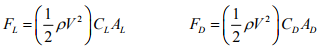Here, ρ is the air or fluid density, V is the speed of the fluid, and AL and AD are measures of the area of the object. Various measures of area are used in practice – when you look up values for drag coefficients you have to check what’s been used. The object’s total surface area could be used. Vehicle manufacturers usually use the projected frontal area (equal to car height x car width for practical purposes) when reporting drag coefficient. CL and CD are dimensionless, so they have no units.

The drag and lift coefficients are not constant, but depend on a number of factors, including:

1. The shape of the object
2. The object’s orientation relative to the flow (aerodynamicists refer to this as the `angle of attack’)
3. The fluid’s viscosity η , mass density ρ , flow speed V and the object’s size. Size can be quantified by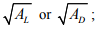other numbers are often used too. For example, to quantify the drag force acting on a sphere we use its diameter D. Dimensional analysis shows that CD and CL can only depend on these factors through a dimensionless constant known as `Reynold’s number’, defined as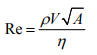For example, the graph on the right shows the variation of drag coefficient with Reynolds number for a smooth sphere, with diameter D. The projected area AD = πD2/4 was used to define the drag coefficient

Many engineering structures and vehicles operate with Reynolds numbers in the range 103 − 106 , where drag coefficients are fairly constant (of order 0.01 - 0.5 or so). Lift coefficients for most airfoils are of order 1 or 2, but can be raised as high as 10 by special techniques such as blowing  air over the wing)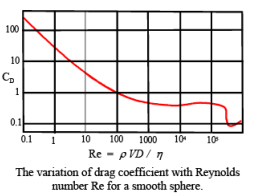Lift and drag forces are of great interest to aircraft designers. Lift and drag forces on an airfoil are computed using the usual formula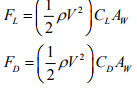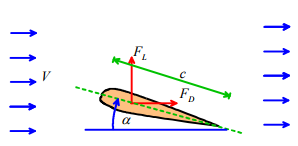The wing area AW = cL where c is the chord of the wing (see the picture) and L is its length, is used in defining both the lift and drag coefficient.

The variation of CL and CD with angle of attack α are crucial in the design of aircraft. For reasonable values of α (below stall - say less than 10 degrees) the behavior can be approximated by

CL = kLα

CD = kDp +kDlα2

where kL , kDp and kDI are more or less constant for any given airfoil shape, for practical ranges of Reynolds number. The first term in the drag coefficient, kDp , represents parasite drag – due to viscous drag and some pressure drag. The second term kDlα2 is called induced drag, and is an undesirable byproduct of lift.

The graphs on the right, (taken from `Aerodynamics for Naval Aviators, H.H. Hurt, U.S. Naval Air Systems Command reprint’) shows some experimental data for lift coefficient CL as a function of AOA (that’s angle of attack, but you’re engineers now so you have to talk in code to maximize your nerd factor.  That’s NF).  The data suggest that kL ≈ 0.1deg −1 , and in fact a simple model known as `thin airfoil theory’ predicts that lift coefficient should vary by 2π per radian (that works out as 0.1096/degree)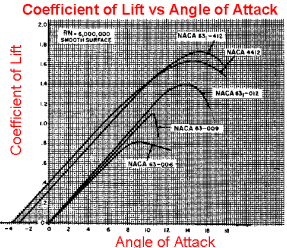The induced drag coefficient kDI can be estimated from the formula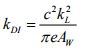where kL ≈ 0.1 deg-1, L is the length of the wing and c is its width; while e is a constant known as the `Oswald efficiency factor.’  The constant e is always less than 1 and is of order 0.9 for a high performance wing (eg a jet aircraft or glider) and of order 0.7 for el cheapo wings.

The parasite drag coefficient kDp is of order 0.05 for the wing of a small general aviation aircraft, and of order 0.005 or lower for a commercial airliner.

Interatomic forces

Engineers working in the fields of nanotechnology, materials design, and bio/chemical engineering are often interested in calculating the motion of molecules or atoms in a system.

They do this using `Molecular Dynamics,’ which is a computer method for integrating the equations of motion for every atom in the solid.  The equations of motion are just Newton’s law – F=ma for each atom – but for the method to work, it is necessary to calculate the forces acting on the atoms.  Specifying these forces is usually the most difficult part of the calculation.

The forces are computed using empirical force laws, which are either determined experimentally, or (more often) by means of quantummechanical calculations.  In the simplest models, the atoms are assumed to interact through pair forces.   In this case

• The forces exerted by two interacting atoms depends only on their relative positions, and is independent on the position of other atoms in the solid
• The forces act along the line connecting the atoms.
• The magnitude of the force is a function of the distance between them.  The function is chosen so that (i) the force is repulsive when the atoms are close together; (ii) the force is zero at the equilibrium interatomic spacing; (iii) there is some critical distance where the attractive force has its maximum value (see the figure) and (iv) the force drops to zero when the atoms are far apart.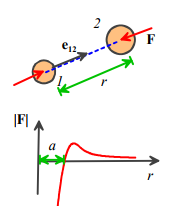Various functions are used to specify the detailed shape of the force-separation law. A common one is the so-called ‘Lennard Jones’ function, which gives the force acting on atom (1) as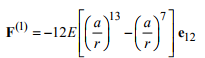Here a is the equilibrium separation between the atoms, and E is the total bond energy – the amount of work required to separate the bond by stretching it from initial length a to infinity.

This function was originally intended to model the atoms in a Noble gas – like He or Ar, etc. It is sometimes used in simple models of liquids and glasses. It would not be a good model of a metal, or covalently bonded solids. In fact, for these materials pair potentials don’t work well, because the force exerted between two atoms depends not just on the relative positions of the two atoms themselves, but also on the positions of other nearby atoms. More complicated functions exist that can account for this kind of behavior, but there is still a great deal of uncertainty in the choice of function for a particular material.

Offer running on EduRev: Apply code STAYHOME200 to get INR 200 off on our premium plan EduRev Infinity!

## Introduction to Dynamics and Vibrations- Notes, Videos, MCQs

20 videos|53 docs

,

,

,

,

,

,

,

,

,

,

,

,

,

,

,

,

,

,

,

,

,

;